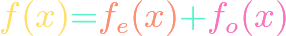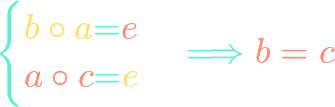# Algebra## Unique Even and Odd Parts of a Function

https://www.youtube.com/watch?v=RRcRDVCA7Bg Even functions $f$ are symmetric across the $y$-axis, meaning $f(-x)=f(x)$. Odd functions $g$ are symmetric across the origin, meaning $g(-x)=-g(x)$. As long as the domain of a function is symmetric across $0$ on the real line, it turns out that the function can be uniquely written as a sum of an even function and …## Inverses in a Binary Operation are Unique

Adding a number to its negative produces $0$. Multiplying a non-zero number by its reciprocal produces $1$. We show that, given a fixed element that has an inverse in an associative binary operation (like addition or multiplication), the inverse is unique. So a number cannot have more than one negative or more than one reciprocal.